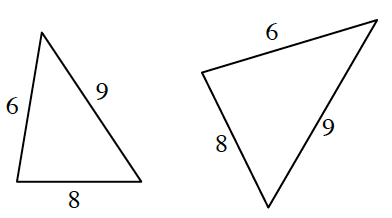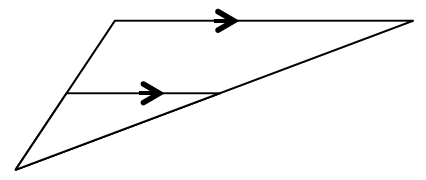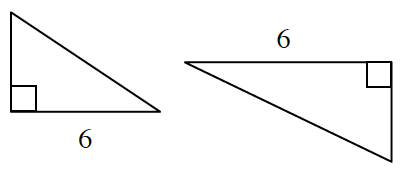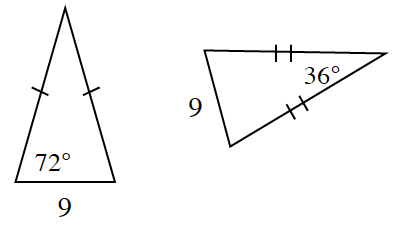### Home > INT1 > Chapter 7 > Lesson 7.1.6 > Problem7-68

7-68.

For each pair of triangles below, decide whether the triangles are congruent. Justify each conclusion with a flowchart.

1.Compare the ratios of each of the corresponding sides of the two triangles. What has to be true about these ratios for the triangles to be congruent?

1.The two triangles are similar. Justify this by examining the angles of each triangle. Is it possible for these triangles to be congruent?

1.There is not enough information to determine whether or not the two triangles are similar.

1.Both triangles are isosceles. Use this information and the Triangle Sum Theorem to find the values of the unknown angles.